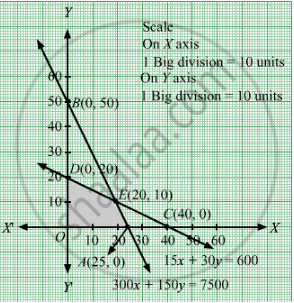# One Kind of Cake Requires 300 Gm of Flour and 15 Gm of Fat, Another Kind of Cake Requires 150 Gm of Flour and 30 Gm of Fat. - Mathematics

Sum

One kind of cake requires 300 gm of flour and 15 gm of fat, another kind of cake requires 150 gm of flour and 30 gm of fat. Find the maximum number of cakes which can be made from 7.5 kg of flour and 600 gm of fat, assuming that there is no shortage of the other ingradients used in making the cake. Make it as an LPP and solve it graphically.

#### Solution

Let there be x cakes of first kind and y cakes of second kind.

∴ x ≥ 0 and y ≥ 0

The given information can be compiled in the form of a table as:

 Flour (gm) Fat (gm) Cakes of first kind 300 15 Cakes of second kind 150 30 Availability 7500 600

Therefore, constraints are:

$300x + 150y \leq 7500$
$15x + 30y \leq 600$

Total number of cakes that can be made = Z = x + y

Thus  , the  given problem  can be mathematically formulated as :

Maximize z = x + y

subject to constraints ,

300x + 150y  ≤ 7500

15x + 30 y ≤ 600

xy ≥ 0

First we will convert inequations into equations as follows:
300x + 150y = 7500, 15x + 30y = 600, x = 0 and y = 0

Region represented by 300x + 150y ≤ 7500:
The line 300x + 150y = 7500 meets the coordinate axes at A(25, 0) and $B\left( 0, 50 \right)$  respectively. By joining these points we obtain the line 300x + 150y = 7500. Clearly (0,0) satisfies the 300x + 150y = 7500. So, the region which contains the origin represents the solution set of the inequation 300x + 150y ≤ 7500.

Region represented by 15x + 30y ≤ 600:
The line 15x + 30y = 600 meets the coordinate axes at C(40, 0) and $D\left( 0, 20 \right)$ respectively. By joining these points we obtain the line 15x + 30y = 600. Clearly (0,0) satisfies the inequation 15x + 30y ≤ 600. So,the region which contains the origin represents the solution set of the inequation 15x + 30y ≤ 600.

Region represented by ≥ 0 and y ≥ 0:
Since, every point in the first quadrant satisfies these inequations. So, the first quadrant is the region represented by the inequations x ≥ 0, and y ≥ 0.
The feasible region determined by the system of constraints 300x + 150y ≤ 7500, 15x + 30y ≤ 600, x ≥ 0 and y ≥ 0 are as follows.The corner points are O(0, 0), A(25, 0), E(20, 10) and D(0, 20).

The values of Z at these corner points are:

 Corner points Z = x + y O(0, 0) 0 A(25, 0) 25 E(20, 10) 30 D(0, 20) 20

Thus, maximum numbers of cakes that can be made from 20 of the first kind and 10 of the other kind.

Concept: Graphical Method of Solving Linear Programming Problems
Is there an error in this question or solution?

#### APPEARS IN

RD Sharma Class 12 Maths
Chapter 30 Linear programming
Exercise 30.3 | Q 9 | Page 39